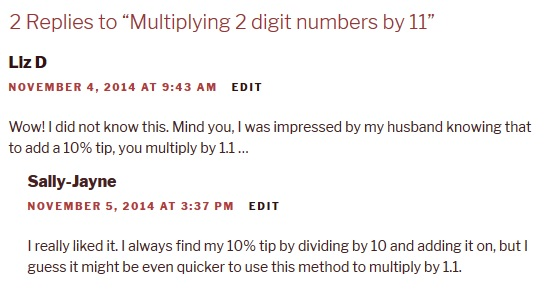## Multiplying 2 digit numbers by 11

Earlier this year I discovered a trick for multiplying 2 digit numbers by 11.  I taught this to some of the children I tutor to help them work out 11×11 and 11×12 which they had been struggling with but then as it works for all 2 digit numbers they have enjoyed baffling their family and friends with their ability to perform calculations such as 54 x 11 in their head.

Apparently it’s not as big a secret as I had thought (well, everyone in my family already knew how), but I’m going to share it anyway just in case some of you don’t already know how…

First of all you split the digits of the two digit number, and these become the first and last digits of the answer. Eg with 54 x 11, the answer is going to start with 5 and end with 4. To get the number in the middle you just add the two digits together. 5 + 4 = 9, so put 9 in the middle. Your answer is 594.

35 x 11 = 3 [3+5] 5   that is 385
42 x 11 = 4 [4+2] 2   that is 462.

That’s all very well, but what if the two digits add up to more than 9?  That’s easy too. You partition that number, the units become the middle digit and the tens get added to the first digit.

86 x 11 = 8 [8+6] 68  6 → uh oh!  It can’t be 8146. That’s ok – split the 14. The 4 becomes the middle digit, and the ten (1) gets added to the first digit → 8+1=9, so the answer is 946.

Happy calculating!